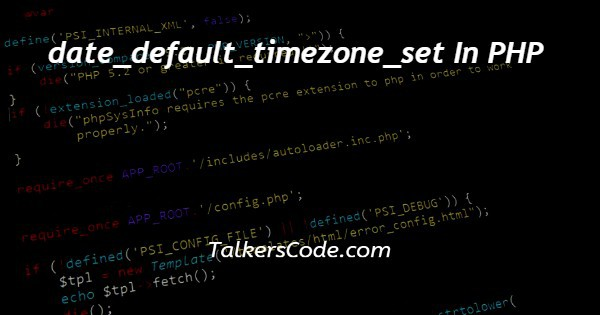# date_default_timezone_set In PHP

Last Updated : Jan 1, 2023In this tutorial we will show you the solution of date_default_timezone_set in PHP, today we will learn about date_default_timezone_set in php which is an inbuilt function of php.

Now, let us see what the inbuilt function is and why it is used, etc. Here, below we are going to show you two ways to use this function. let us see the below examples for better understanding.

## Step By Step Guide On date_default_timezone_set In PHP :-

The date_default_timezone_set() function sets the default time zone used by all date/time functions in the script.

The function returns true if time zone is valid otherwise it return false. Now, let us see or go through th syntax of this function.

bool date_default_timezone_set( \$timezone_identifier )

We can also use this function as below also

date_default_timezone_set(timezone)

Now, we can use this function on two ways. let us see both of them.

Here, we below see the first way to use this function.

```<?php
date_default_timezone_set("Asia/New Delhi");
echo date_default_timezone_get();
// prints the output of above function
?>```

The code given below is second way to use date_default_timezone_set() in php.

```<?php
date_default_timezone_set('America/NewYork');
// output of this function is stored to a variable named timezone
\$timezone=date_default_timezone_get();
// compares the timezone with ini-set function here
if(strcmp(\$timezone,ini_get('date.timezone')))
{
echo 'Script timezone differs from ini-set timezone here.';
}
else
{
echo 'Script timezone and ini-set timezone match here.';
}
?>```
1. Here, in first example we simply use the date_default_timzone_set() function inside the basic php structure.
2. Whereas the <?php is opening tag of php and ?> is closing tag. All the data or say code of php is always written under or inside these tags.
3. Here, we use the function and pass required timezone as parameter. Then echo this function to display the time zone. This is first use of this date_default_timezone_set() function.
4. In another example, we again create basic php structure and then use this function. Inside this we pass parameters that is time zone required.
5. After this we store the output of this function inside a variable named as time zone. You can see the after example for better understanding.
6. Now, our next task is to check this value of stored variable to using ini.get function.
7. Here, as you see that we use if condition to check weather time zone matches or not. If the time zone matches then the code inside else display otherwise code given inside if executed.
8. Now, let us talk about if condition. Here we see that we use strcmp function and parameter given is first in which we store the value of function whereas second one is time from ini.set function.
9. The output of this function is based on decision of condition.

## Conclusion :-

At last in conclusion, here we can say that with the help of this article we are able to understand about date_default_timezone_set in php.

I hope this tutorial on date_default_timezone_set in PHP helps you and the steps and method mentioned above are easy to follow and implement.